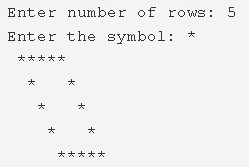# C Program Hollow Mirrored Rhombus Star Pattern | C Programs

C Program to print a Hollow mirrored Rhombus Star Pattern – In this article, we will discover how to print a hollow mirrored rhombus star pattern in various ways in C programming.

Suitable examples and sample programs have also been added so that you can understand the whole thing very clearly. The compiler has also been added with which you can execute it yourself.

The ways mentioned in this piece are as follows:

• Using For Loop
• Using While Loop
• Using Do-While Loop

As we all know, a rhombus is a two-dimensional quadrilateral in the field of geometry.

A rhombus, as we all know, has equal sides and the opposite sides are parallel. The opposite angles are also equal in nature.

The diagonals of a rhombus cut each other at right angles.

A rhombus is basically a parallelogram where two adjacent sides are equal.As you can see, we have to first enter the number of rows. Depending on that, the rhombus can be printed using the symbol “*”.

Thus, the various methods used to print a hollow mirrored rhombus star pattern are as follows:

## Using For Loop

1. Read the no.of rows, entered the symbol and store the values into the variables n, ch.

2) The outer for loop iterates through rows with the structure for(i=1;i<=n;i++).

3) The 1st inner for loop iterates through columns with the structure for(j=i;j>0;j–).

a) Prints space.

4) For 1st row,nth row(i=1 or n) the 2nd inner for loop iterates through columns with the structure for(j=1;j<=n;j++).

a) Prints symbol.

5) For i!=1 or i!=n(other than 1st row,last row)

The 3rd inner for loop iterates through columns with the structure for(j=1;j<=n;j++)

a) Prints symbol if j=1 or n

b) Otherwise it prints space.

6) For each iteration of the outer loop, cursor comes to next line.

Output:

### Using While Loop

1. The outer while loop iterates through rows until the condition i<=n becomes false.

2) The 1st inner while loop iterates through columns and prints space until the condition –j>0 becomes false.

3) For 1st row, nth row(i=1 or i=n)

The 2nd inner while loop iterates through columns and prints symbol until the condition j<=n becomes false.

4) For i!=1 or i!=n(other than 1st row,nth row)

The 3rd inner while loop iterates through columns until the condition j<=n becomes false.

a) Prints symbol if j=1 or j=n.

b) Otherwise prints space.

5) For each iteration of the outer while loop, the cursor comes to next line.

Output:

#### Using Do-While Loop

1. The outer do-while loop iterates through rows.

2) The 1st inner do-while loop iterates through columns.

a) Prints space.

b) j value decreased by 1.

c) Checks the condition –j>0. If the condition is true then loop iterates again.Repeats until the condition becomes false.

3) For 1st row, nth row.

The 2nd inner do-while loop iterates through columns.

a) Prints symbol if j=1 or n.

b) Otherwise prints space.

c) j value increased by 1.

d) Checks the condition ++j<=n.If the condition is true loop iterates again.Repeats until the condition ++j<=n becomes false.

4) Cursor comes to next line.

5) i value increased by 1.Checks the condition i<=n. If the condition is true then outer loop iterates again. Repeats until the condition i<=n becomes false.

Output:
x

## C Program To Check If Alphabet, Digit or Special Character | C Programs

C program to check for an alphabet, digit or special character – In this article, ...